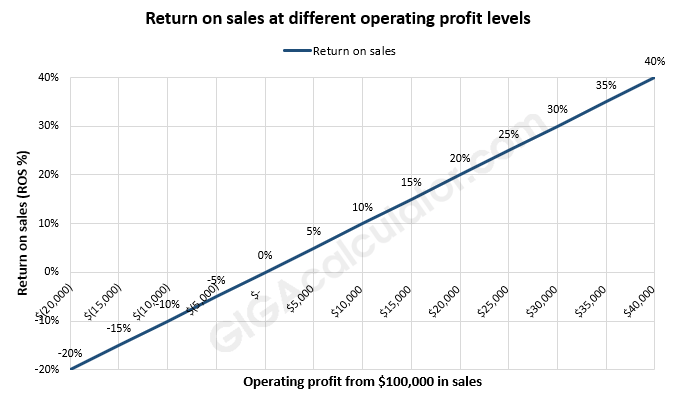# Return on Sales Calculator

Use this calculator to easily calculate ROS (return on sales) based on the net profit and the total value of the sales that generated it.

\$
\$
Share calculator:

Embed this tool:
get code

## ROS Formula

The formula for ROS used in our return on sales calculator is simple:

Return on Sales = Operating Profit / Net Sales x 100

Operating profit is also known as operating income in the U.K.. Both input values are in the relevant currency while the result is a ratio which is then converted to a percentage by a simple multiplication by 100.

The equation does not account for non-operating activities and expenses like taxes, interest payments, and others. Consequently our ROS calculator doesn't as well.

## How to calculate return on sales?

Return on Sales (ROS) is a metric used to estimate what percentage of sales are converted to profit and measures the efficiency and profitability of business ventures after accounting for all costs. It is calculated as the company's net profit relative to the total value of its sales.

Using the above formula, one needs to simply substitute the relevant values and use a calculator to arrive at the final value. For example, if the operating profit (income) of a company is \$100,000 and it required sales worth \$1,000,000 to generate it, its return on sales 10%. In other words, it makes 1 dollar of profit for every 10 dollars in sales.Obviously, a positive ROS means the company is making a profit while a negative ROS means it is registering losses. In the chart above, an operating profit below zero results in negative returns.

## What is a good return on sales?

In most mature businesses with a low level of risk a 5% annual return on sales is considered pretty decent. Highly-volatile and risky investments, however, would be expected to have a return on sales of 10%, 20% or even higher return to justify the risk-adjusted cost. In the end, the same output from our ROS calculator will be "good" for some and "bad" for others, depending on circumstances such as the niche, competition, perceived risk, and investor time preference, among others.

The way improve your return on sales is to either successfully cut costs or increase the operating revenue, or both. Of course, these measures need to be evaluated for both short and long-term effects since these can be very different.

#### Cite this calculator & page

If you'd like to cite this online calculator resource and information as provided on the page, you can use the following citation:
Georgiev G.Z., "Return on Sales Calculator", [online] Available at: https://www.gigacalculator.com/calculators/return-on-sales-calculator.php URL [Accessed Date: 06 Jun, 2023].